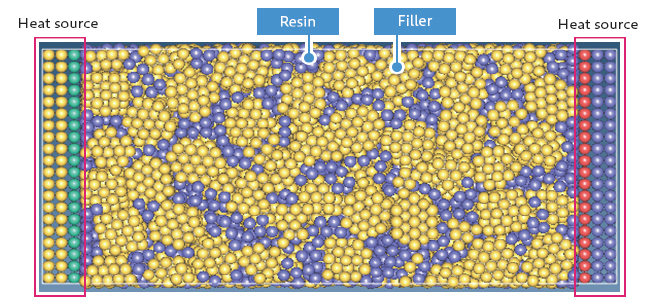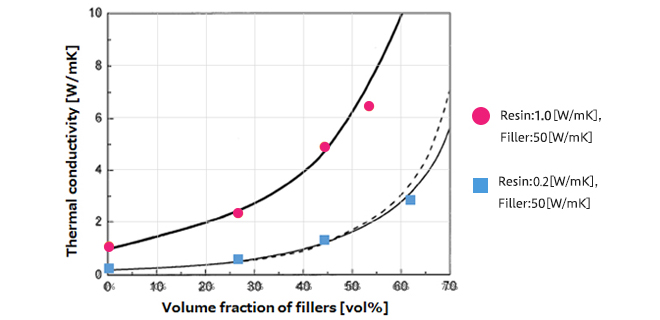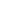Continuum model
Thermal
Materials Science

# [Analysis Example] Thermal conductivity calculations for filler resin composites

Heat conduction simulation using Particle method

## Objectives and Methods

In recent years, heat generation in electronic equipment has become a problem due to the high integration of electronic circuits. Resin materials with high thermal conductivity are needed to efficiently dissipate heat from circuits. However, the thermal conductivity of resins used in circuits is generally low, and composite materials in which fillers with high thermal conductivity are mixed with resins have been developed . This case study presents an example of thermal conductivity evaluation of composite materials.

VSOP-PS, one of the engines of J-OCTA, solves the heat conduction equation using the particle method (MPS = Moving Particle Simulation (or Semi-Implicit) method). Compared to other methods, this method has the advantage of being more adaptable to calculations for high-density filled structures, as shown in the figure. The model has three layers at both ends as heat sources, with filler and resin particles in between. The resin is a single particle and the filler is a combination of multiple particles. The effective thermal conductivity is calculated from Fourier's equation using the temperature gradient formed and the thickness of the filled particle layer in the gradient direction.Figure1 : Model

## Results

Figure 2 shows the relationship between the volume fraction of filler and effective thermal conductivity in the resin particle/filler composite modeled in Figure 1. The lines are the results obtained from Bruggeman's approximation . The VSOP-PS results are colored circles and squares. They are close to Bruggeman's approximation. The calculation time for one point is about 30 minutes (about 20,000 particles).Figure2 : Volume fraction dependence of thermal conductivity